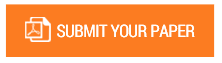Numerical Study of Natural Air Convection in a Domain Bounded by Two Rectangular Parallelepipeds of Square Straight Sections for Ra = 106 for a Value of the Hartmann Number Ha = 0.75

Authors : Aly DIOUF; Omar Ngor THIAM; Oumar DRAME; Mamadou lamine SOW.

Volume/Issue : Volume 7 - 2022, Issue 12 - December

Scribd : https://bit.ly/3COARRZ

DOI : https://doi.org/10.5281/zenodo.7542624

In this numerical simulation, we have proposed to study the natural convection of air in a bounded domain between two rectangular parallelepipeds of square straight sections for a Rayleigh value Ra = 106 . The internal cavity is subjected to a heat flow of constant density while the external cavity is kept at a constant temperature. The air fluid is subjected to a magnetic field oriented at an angle θ = 45° with respect to the horizontal. To overcome the term pressure in the equation of motion, we have used the vorticity formalism as a function of current. The equations obtained were discretized with the finite volume method and were solved by the SIMPLE algorithm. The results obtained through the commercial calculation code Ansys Fluent, in the absence of the magnetic field, show us, for isothermal lines, a stable flow which is manifested by high and low temperatures and for current lines the formation of two recirculation zones of high intensity and rotating in opposite directions. When applying the magnetic field with a Hartmann number Ha = 0.75, we have noted the formation of four recirculation zones for current lines and standardization for isothermal lines.

Keywords : Ansys Fluent; Convection; Hartmann; Rayleigh Number; Finite Volume.

#### CALL FOR PAPERS

Paper Submission Last Date
31 - March - 2023